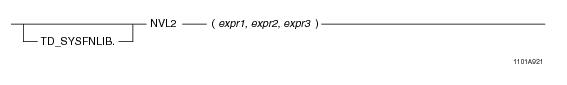# 15.00 - NVL2 - Teradata Database

## Teradata Database SQL Functions, Operators, Expressions, and Predicates

Product
Release Number
15.00
Content Type
Programming Reference
Publication ID
B035-1145-015K
Language
English (United States)

## NVL2

### Purpose

Returns one of two values based on whether or not expr1 is NULL.

### Syntaxwhere:

 Syntax element … Specifies … TD_SYSFNLIB the name of the database where the function is located. expr1 a numeric or character expression. If expr1 is not NULL, expr2 is returned. If expr1 is NULL, expr3 is returned. expr2 a numeric or character expression. expr3 a numeric or character expression.

### ANSI Compliance

This is a Teradata extension to the ANSI SQL:2011 standard.

### Invocation

NVL2 is an embedded services system function. For information on activating and invoking embedded services functions, see “Embedded Services System Functions” on page 24.

### Argument Types and Rules

Expressions passed to this function must have one of the following data types:

BYTEINT, SMALLINT, INTEGER, BIGINT, DECIMAL/NUMERIC, FLOAT/REAL/DOUBLE PRECISION, NUMBER, CHAR, VARCHAR

expr2 and expr3 must be the same data type or else the types must be compatible.

### Result Type

NVL2 is a scalar function whose return data type depends on the data types associated with the arguments passed to the function.

• If expr2 and expr3 are numeric types, the function determines which argument has the highest precedence, converts the other argument to that data type, and returns that data type. For details about the order of precedence, see “Compatible Types” in SQL External Routine Programming.
• If that data type is DECIMAL/NUMERIC and the precision and scale of the two arguments are different, the precision and scale of the return type will be set to achieve the maximum precision possible. For example, if the input arguments are DECIMAL(6,3), DECIMAL(7,4), and DECIMAL(8,7), the return type would need three digits to the left of the decimal point and seven digits to the right of the decimal point to avoid any reduction in precision. In this case, the return data type is set to DECIMAL(10,7)

In cases where it is not possible to maintain the maximum precision, the data will be rounded according to the DBS Control Record RoundHalfWayMagUp field. For example, if the two arguments are DECIMAL(32, 8) and DECIMAL(30, 28), the return type will be DECIMAL(38,14). This will allow for 24 digits to the left of the decimal point (required for the DECIMAL(32,8) argument), and 14 digits to the right of the decimal point.

If the data type is fixed point NUMBER and the precision is less than or equal to 38, the precision and scale of the return type are calculated with the same method used for DECIMAL/NUMERIC. However, if the precision is greater than 38, the return type is changed to NUMBER(*) to avoid loss of accuracy. If the data type is floating point NUMBER, the return type is NUMBER(*).

• If expr2 and expr3 are character types, the function converts the second argument to the data type of the first argument and returns the type as VARCHAR.
• You can also pass arguments with data types that can be converted to the above types using the implicit data type conversion rules that apply to UDFs.

Note: The UDF implicit type conversion rules are more restrictive than the implicit type conversion rules normally used by Teradata Database. If an argument cannot be converted to the required data type following the UDF implicit conversion rules, it must be explicitly cast.

For details, see “Compatible Types” in SQL External Routine Programming.

### Example

The following query:

`SELECT NVL2('England', 'France', 'Spain'); `

returns the second argument, 'France', because the first argument is not NULL.

### Example

The following query:

`SELECT NVL2(NULL, 'France', 'Spain'); `

returns the third argument, 'Spain', because the first argument is NULL.